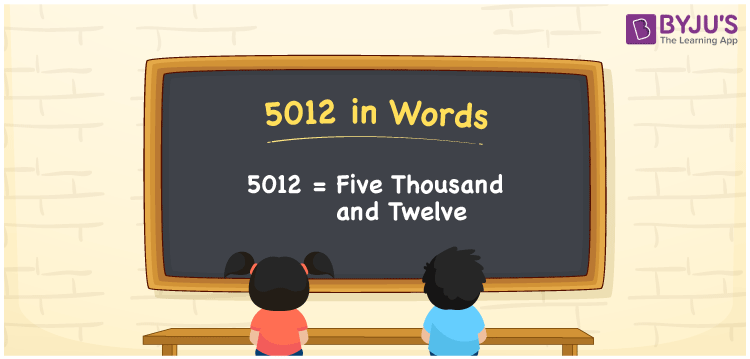# 5012 in words

5012 in words is written as Five Thousand and Twelve. 5012 represents the count or value. The article on Counting Numbers can give you an idea about count or counting. The number 5012 is used in expressions that relate to money, distance, length, year and others. Let us consider an example for 5012. ”The year Five Thousand and Twelve, will be a leap year.”

 5012 in words Five Thousand and Twelve Five Thousand and Twelve in Numbers 5012

## 5012 in English Words## How to Write 5012 in Words?

We can convert 5012 to words using a place value chart. The number 5012 has 4 digits, so let’s make a chart that shows the place value up to 4 digits.

 Thousands Hundreds Tens Ones 5 0 1 2

Thus, we can write the expanded form as:

5 × Thousand + 0 × Hundred + 1 × Ten + 2 × One

= 5 × 1000 + 0 × 100 + 1 × 10 + 2 × 1

= 5012

= Five Thousand and Twelve.

5012 is the natural number that is succeeded by 5011 and preceded by 5013.

5012 in words – Five Thousand and Twelve.

Is 5012 an odd number? – No.

Is 5012 an even number? – Yes.

Is 5012 a perfect square number? – No.

Is 5012 a perfect cube number? – No.

Is 5012 a prime number? – No.

Is 5012 a composite number? – Yes.

## Solved Example

1. Write the number 5012 in expanded form

Solution: 5 × 1000 + 0 × 100 + 1 × 10 + 2 × 1

We can write 5012 = 5000 + 000 + 10 + 2

= 5 × 1000 + 0 × 100 + 1 × 10 + 2 × 1

## Frequently Asked Questions on 5012 in words

Q1

### How to write 5012 in words?

5012 in words is written as Five Thousand and Twelve.
Q2

### Is 5012 a perfect square number?

No. 5012 is not a perfect square number.
Q3

### Is 5012 a prime number?

No. 5012 is not a prime number.